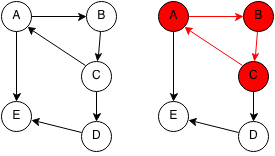## Introduction

A cycle occurs in a graph when a duplicate node is encountered when traversing a tree using a depth first search. In other words, a cycle occurs when you can reach the same node through a path in a graph.

An undirected graph where the number of edges is greater than or equal to the number of nodes will always have cycles.## Implementation

We first mark every node as unvisited. We start at each unvisited node and we do a depth first search from that node. We mark each node along the way as temporarily visited and if we encounter a temporarily visited node, then we have a cycle. After temporarily visiting the nodes, we mark them as visited. If we reach a visited node again, then we stop since we already checked if that node had a cycle.

```public static boolean hasCycle(int[][] adjMatrix) {

// Mark each node as unvisited.
for (int i = 0; i < adjMatrix.length; i++) {
visited[i] = 0;
}
// Check if current node leads to a cycle.
for (int i = 0; i < adjMatrix.length; i++) {
return true;
}
}
return false;
}

public static boolean hasCycleAt(int[][] adjMatrix, int i, int visited[]) {
// If node has been reached again from the starting node, we have a cycle.
if (visited[i] == 1) {
return true;
}
// If node has been permanently visited, we know it was already checked.
if(visited[i] == 2) {
return false;
}

// Mark node as temporarily visited.
visited[i] = 1;

// Iterate through neighbors of current node.
for (int j = 0; j < adjMatrix.length; i++) {